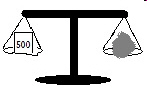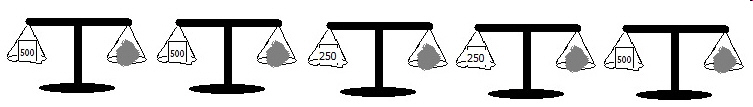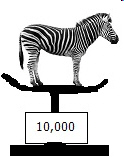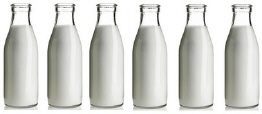3.MD.A.2 Measure and estimate liquid volumes and masses of objects using standa
 Name:    3.MD.A.2 Measure and estimate liquid volumes and masses of objects

Multiple Choice
Identify the choice that best completes the statement or answers the question.

1.

Suzanne had one liter of cola to share with herself and 3 friends.  She began pouring the cola into four cups.  How many millimeters did each girl get?
 a. 250 c. 1000 b. 4 d. 4 ounces

2.

Three friends filled a bucket marked with liters of water to wash a car.   How many milliliters did they put in  the bucket?a. 2.5 c. 2 b. 1 gallon d. 2500

3.The scale says that there are 500 grams of iron on it.  How many kilograms is that?
 a. 0.5 c. 1 lb b. 1 d. 5

4.Each scale measures the iron on the scale in grams.
Read all the scales and tell how many total kilograms of iron are shown.
 a. 500 c. 1 b. 2 d. 5

5.When the vet weighed the zebra above, the scale said 10,000 grams.  How many kilograms did the zebra weigh?
 a. 1000 c. 1 b. 0.5 d. 10

6.a. 5 c. 3 b. 6 d. 12